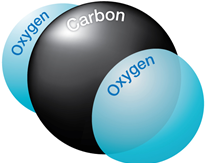## Effect of CO2 on downhole flowrate calculation

Downhole flow rate can be calculated from surface flow rate (stock tank barrels) using the following equation. It is assumed that no gas is dissolved in the water phase and the water formation volume factor is equal to one.

Downhole flow rate = [(Oil rate)sc × Bo] + [(Free GOR) × (Oil rate)sc × Gas FVF] + (Water rate)sc

Free GOR = Producing GOR – Solution GOR, therefore:

## q = ( Qo × Bo ) + [ ( R – Rs) × Qo × Bg × 1000] + Qw

Where:

• q = downhole flow rate (bbl/d or m3/d)
• Qo = Oil flow rate at standard conditions (stb/d or m3sc/d)
• Bo = Oil formation volume factor (bbl/stb or m3sc/m3sc)
• R = Producing gas-oil ratio (scf/stb or m3sc/m3sc)
• Rs = Solution gas-oil ration (scf/stb or m3sc/m3sc)
• Bg = Gas formation vol. factor (bbl/mscf or m3sc/m3sc)
• Qw = Water flow rate at standard conditions (stb/d or m3sc/d)

### Effect of CO2 on downhole flowrate calculation:If CO2 is present, the calculation of downhole flow rate becomes more complex for many reasons: